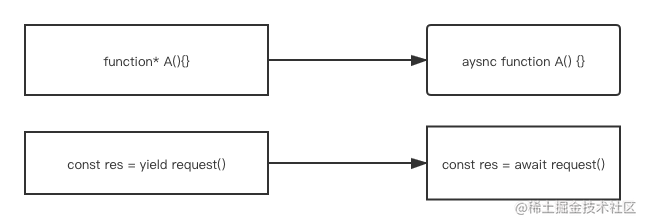# CO源码解读

#### 前言

• 一个五年前的库，在目前的前端开发中并不常见
• 这个库作用于genertor函数，但在genertor在日常的开发中很难使用到，一般都是使用promise和 async等方式

#### co是用来做什么呢#### 用法示例

``````function request(name, ms = 1000) {
return new Promise((resolve) => {
setTimeout(() => {
resolve({name});
}, ms);
});
}

function* getData() {
let name1 = yield request('cc')
let name2 = yield request(name1)
return name2
}

// 按照正常的方式的用法

let data = getData()
let {value} = data.next()
value.then(res => {
let { value } = data.next(res)
value.then(res => {
...
})
})

co(getData()).then(res => {})

#### co源码解析

``````function co(gen) {

var ctx = this; // 保存当前环境下的this
var args = slice.call(arguments, 1); // 保存除了gen的所有变量

return new Promise(function(resolve, reject) {
// 如果gen传入的是一个方法 则调用gen函数并保存返回值
if (typeof gen === 'function') gen = gen.apply(ctx, args);
//如果不存在返回值或者返回值的next不是一个方法 则直接返回gen
if (!gen || typeof gen.next !== 'function') return resolve(gen);
// 先执行一下成功回调
onFulfilled();
// 成功的回调
function onFulfilled(res) {
var ret; // 用来保存gen.next的返回值
try {
ret = gen.next(res);
} catch (e) {
return reject(e); // 如果失败直接报错
}
next(ret); // //把返回结果传入到next函数中
return null;
}

// 失败回调 原理同上
function onRejected(err) {
var ret;
try {
ret = gen.throw(err);
} catch (e) {
return reject(e);
}
next(ret);
}

// 反复调用函数
function next(ret) {
if (ret.done) return resolve(ret.value); // 如果返回值已经是gen最后的节点了、则直接resolve(ret.value)
var value = toPromise.call(ctx, ret.value); //否则就把ret.value的返回值转换成promise
if (value && isPromise(value)) return value.then(onFulfilled, onRejected); // 转换成功后调用then函数继续执行onFulfilled、和onRejected方法
return onRejected(new TypeError('You may only yield a function, promise, generator, array, or object, '
+ 'but the following object was passed: "' + String(ret.value) + '"')); //如果返回值类型不对则直接报错
}
});

#### 结语

co框架的源码地址 github.com/tj/co